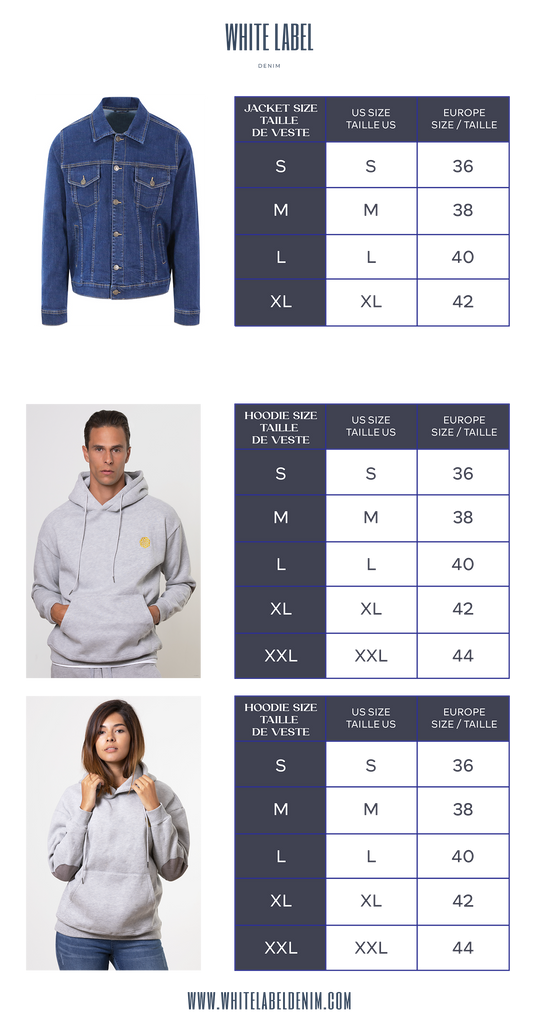# Our Sizing and Key sizes

We are working in European sizes. Please check the documents below to check our measurements charts.

Your order options are divided by a range of 6 sizes as per follow :

For Denim Women styles :

OPTION 1 : 150 pcs - 34 = 20 pcs, 36 = 25 pcs, 38 = 30 pcs, 40 = 30pcs, 42 = 25 pcs, 44 = 20 pcs.

OPTION 2 : 300 pcs - 34 = 40 pcs, 36 = 50 pcs, 38 = 60 pcs, 40 = 60pcs, 42 = 50 pcs, 44 = 20 pcs.

OPTION 3: Bespoke solution - All our sizes divided as per your requirements (must be more than 300pcs)

For Denim Men styles :

OPTION 1 : 150 pcs - 38 = 20 pcs, 40 = 25 pcs, 42 = 32 pcs, 44 = 30pcs, 46 = 25 pcs, 48 = 20 pcs.

OPTION 2 : 300 pcs - 38 = 40 pcs, 40 = 50 pcs, 42 = 60 pcs, 44 = 60pcs, 46 = 50 pcs, 48 = 20 pcs.

OPTION 3: Bespoke solution - All our sizes divided as per your requirements (must be more than 300pcs)

For unisex styles (Men and Women) Hoodies :

OPTION 1 : 100 pcs - S = 15 pcs, M = 30 pcs, L = 30pcs, XL= 15 pcs, XXL = 10 pcs.

OPTION 2 : 200 pcs - S = 30 pcs, M = 60 pcs, L = 60pcs, XL= 30 pcs, XXL = 20 pcs.

For Denim Jackets Women styles :

OPTION 1 : 100 pcs - S = 20 pcs, M = 30 pcs, L = 30 pcs,  XL = 20pcs

OPTION 2 : 200 pcs - S = 40 pcs, M = 60 pcs, L = 60 pcs,  XL = 40pcs

For Denim Jackets Men styles :

OPTION 1 : 100 pcs - S = 20 pcs, M = 30 pcs, L = 30 pcs,  XL = 20pcs

OPTION 2 : 200 pcs - S = 40 pcs, M = 60 pcs, L = 60 pcs,  XL = 40pcsen
• en
• fr